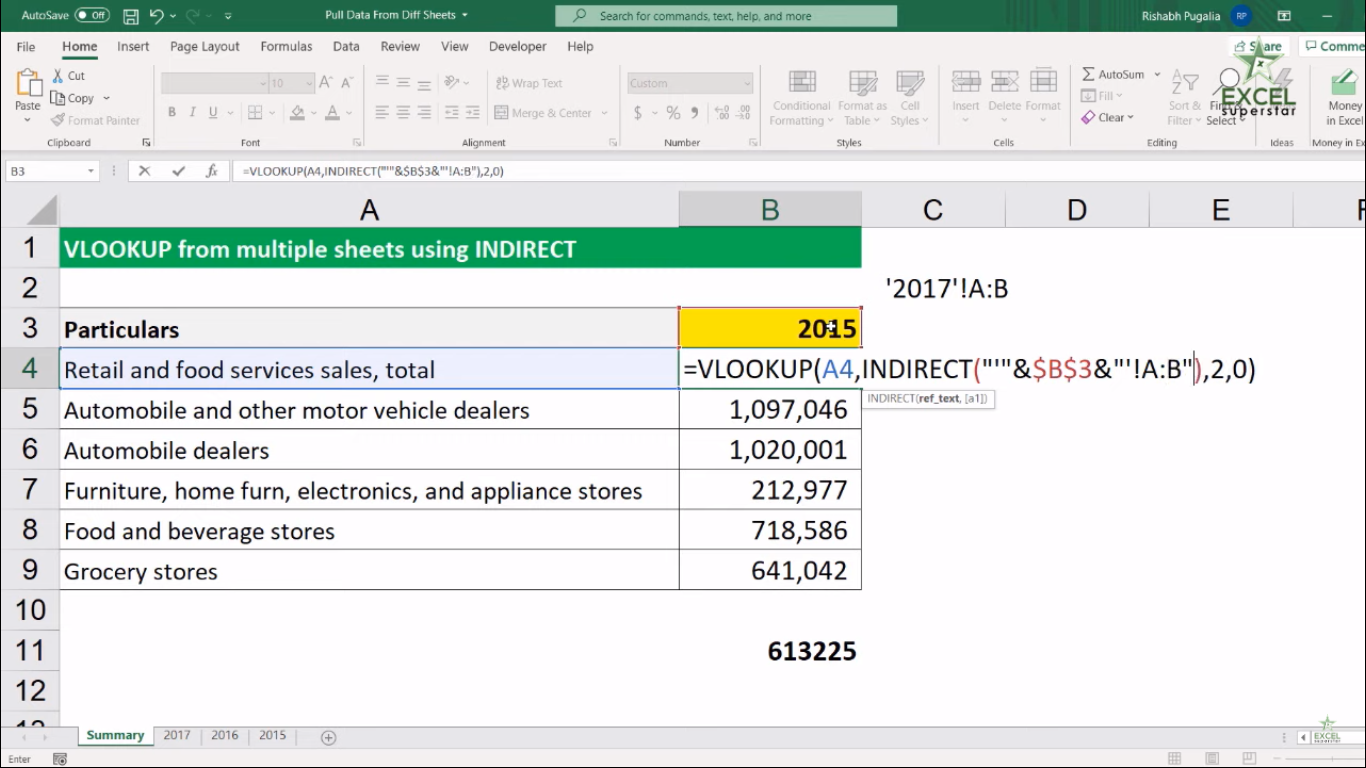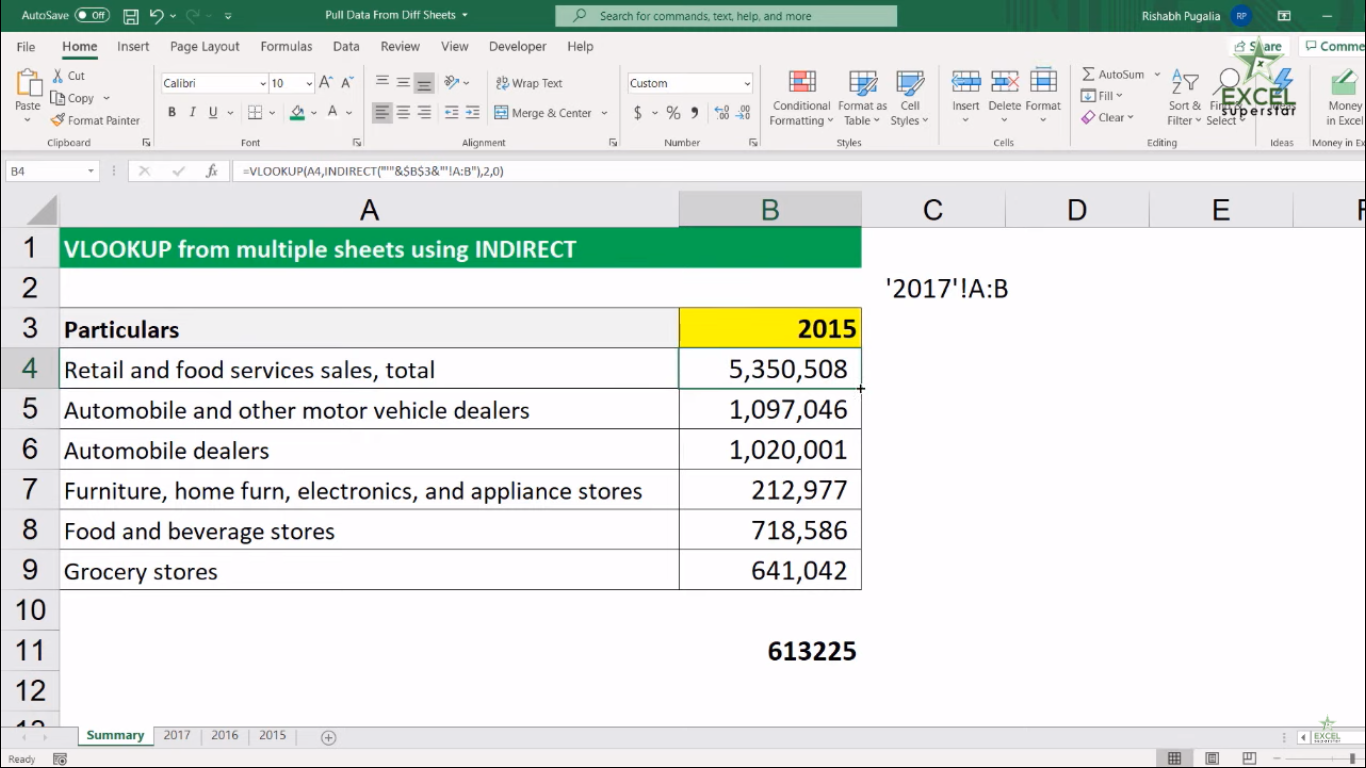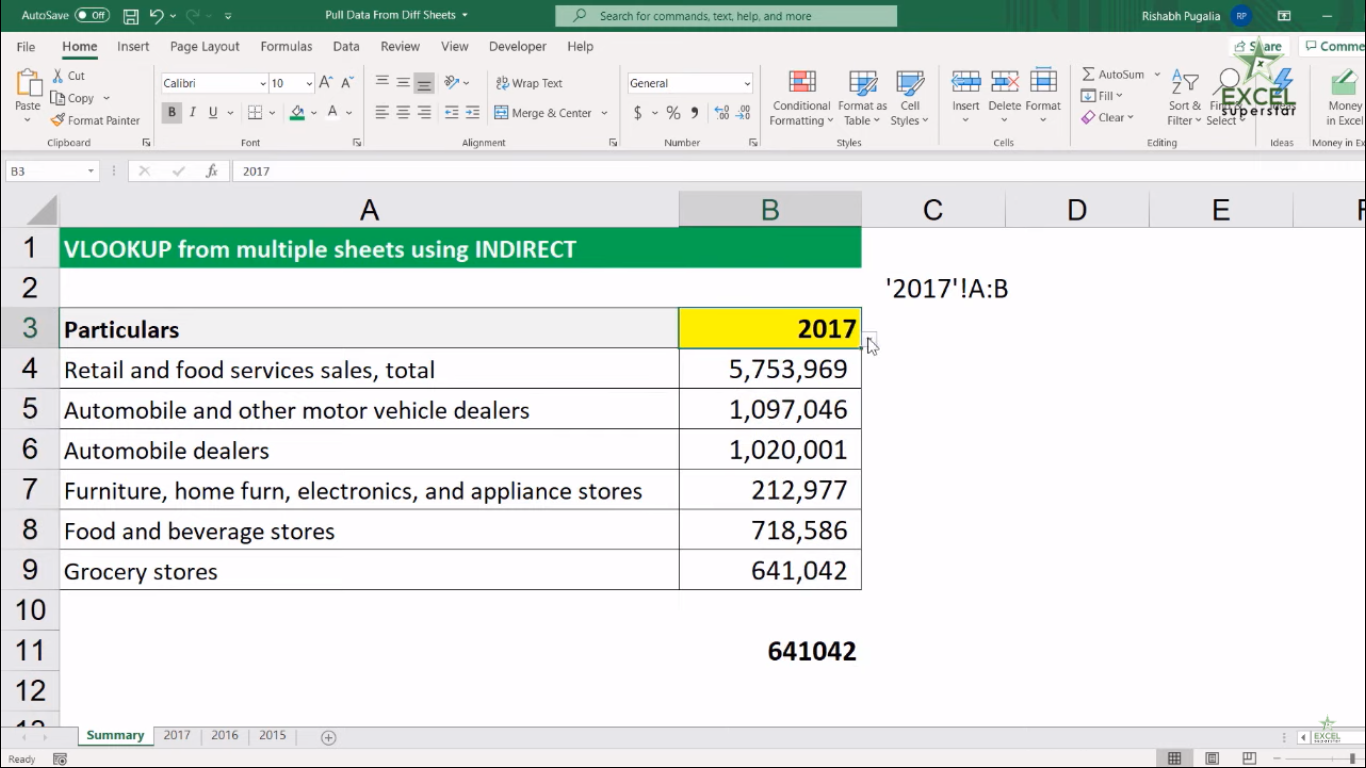# Excel VLOOKUP from Multiple SheetsIn this tutorial, we will learn about How to Use one VLOOKUP to pull data from multiple sheets in Excel.

Example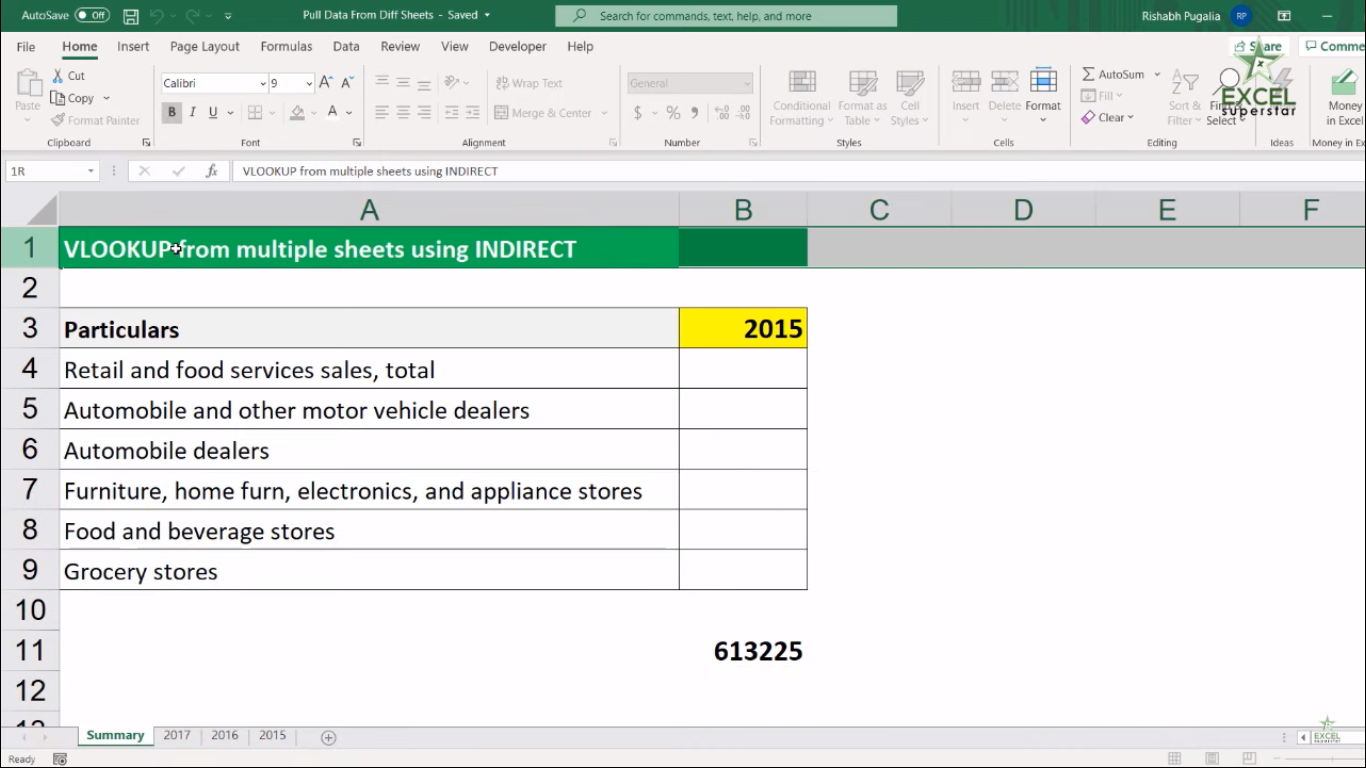Here I have a table which consists of Various Services along with the given year. Now I have three sheets of different years.

2017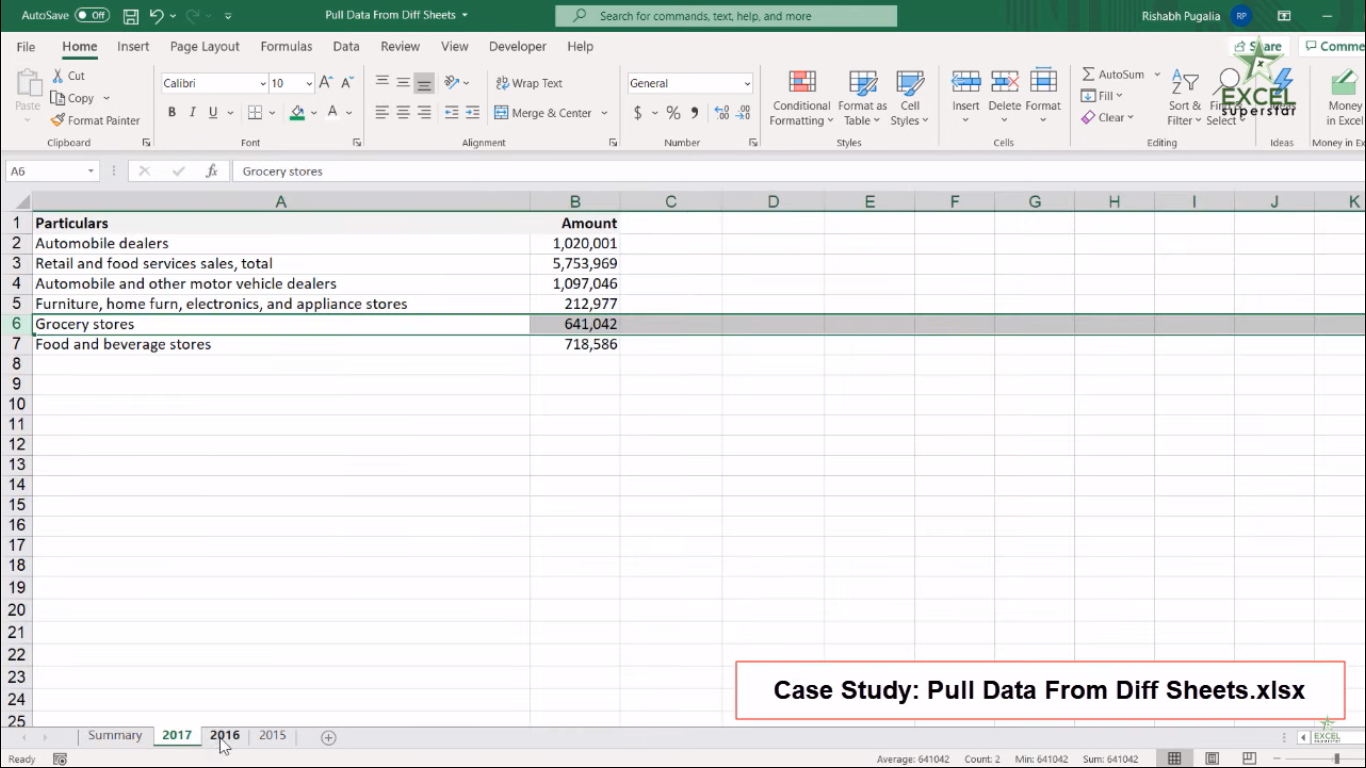2016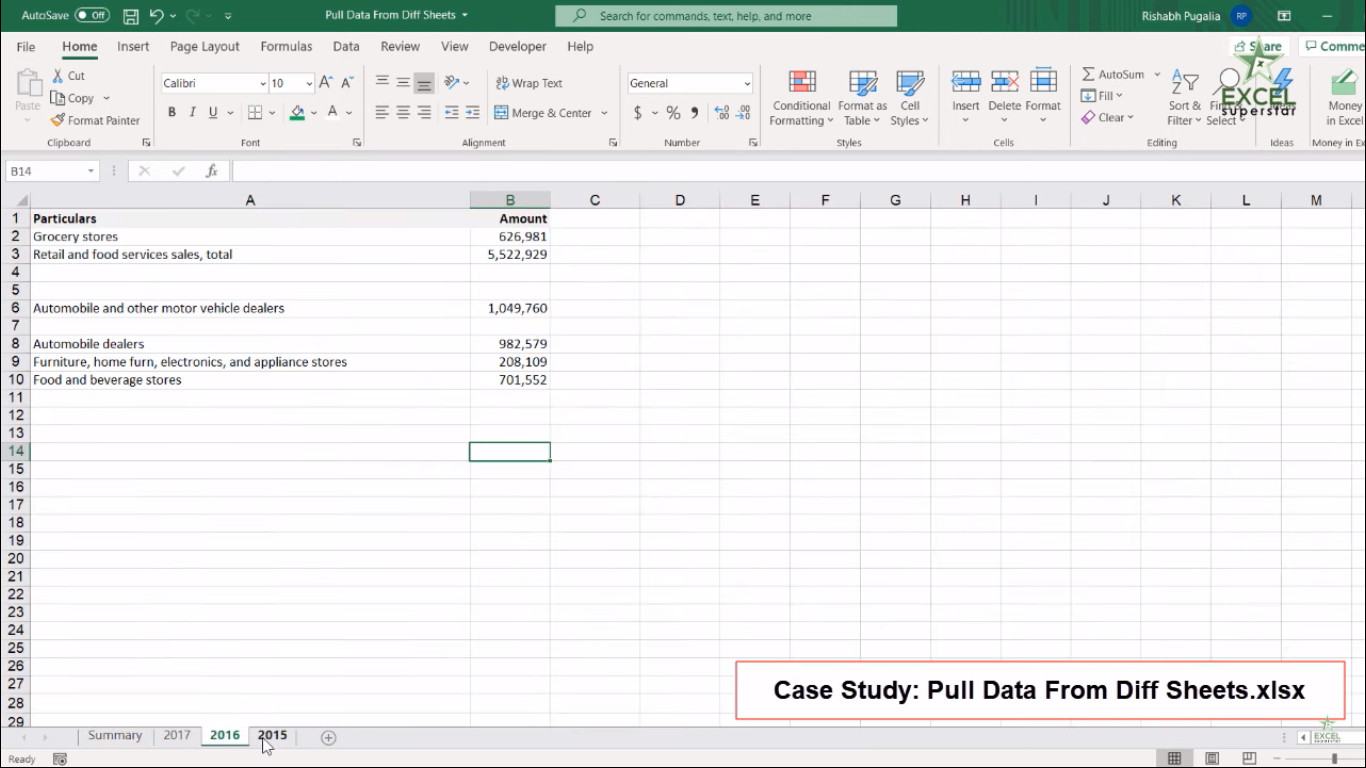2015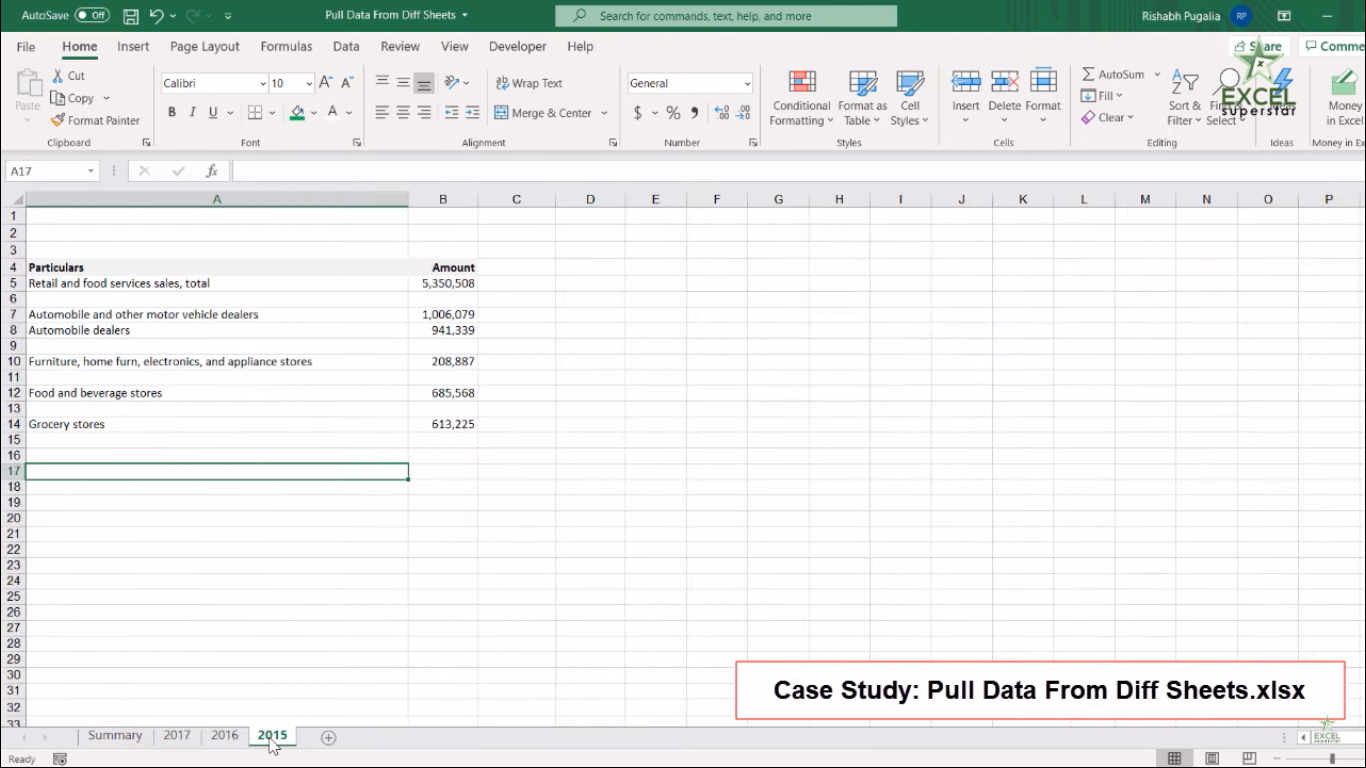In every sheet, there are common items like Grocery Stores, Food, and Beverage Stores. Now the problem is in every sheet; the item Grocery store lies in different rows.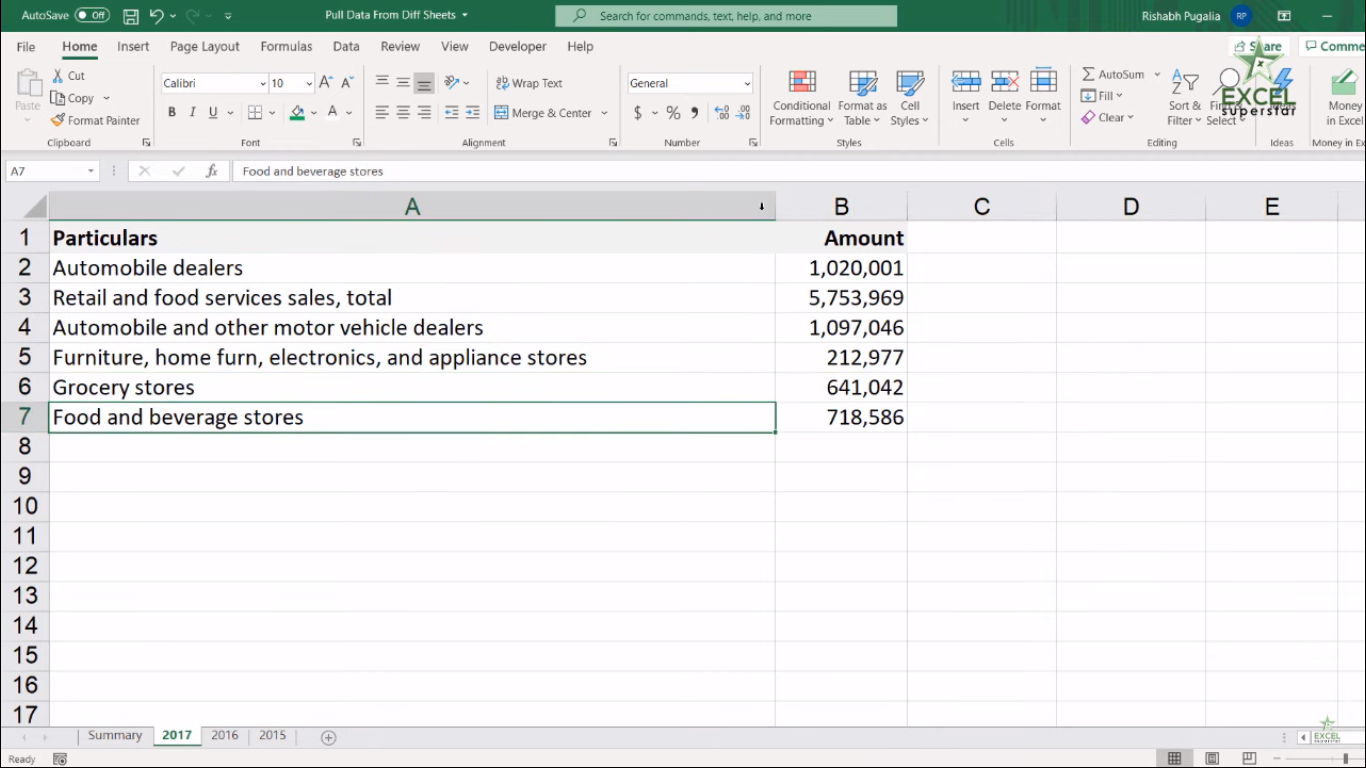So now we want that when we change the year from the given list, then we should get the answer from the given year only.

In order to get the answer of Grocery Store, we will be applying VLOOKUP Formula by applying the following steps given below:

1. Write =VLOOKUP and Press Tab Key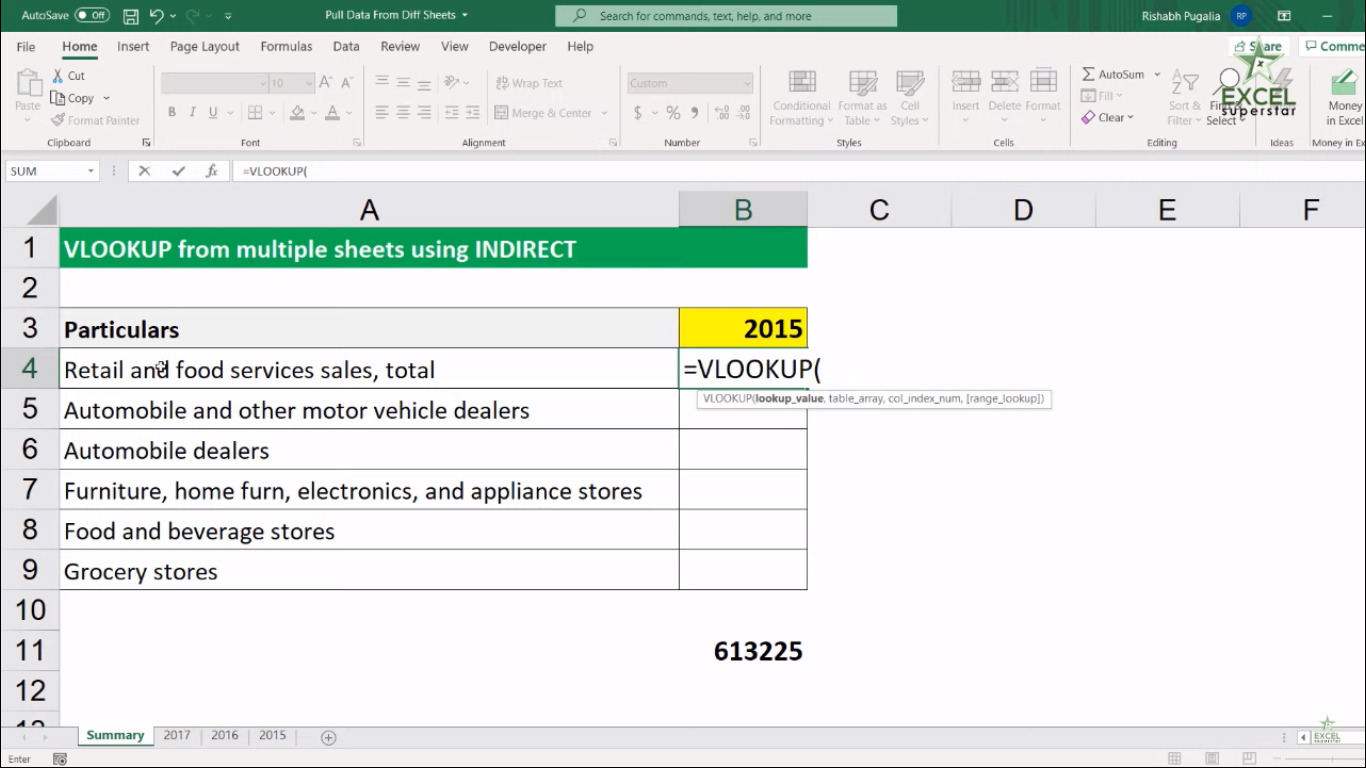Now there are 4 parameters

(lookup_value, table_array, col_index_num, [range_lookup])

The first parameter lookup_value means the value to look for in the first column of a table.

2. Select the Retail and Food Services value =VLOOKUP(A4,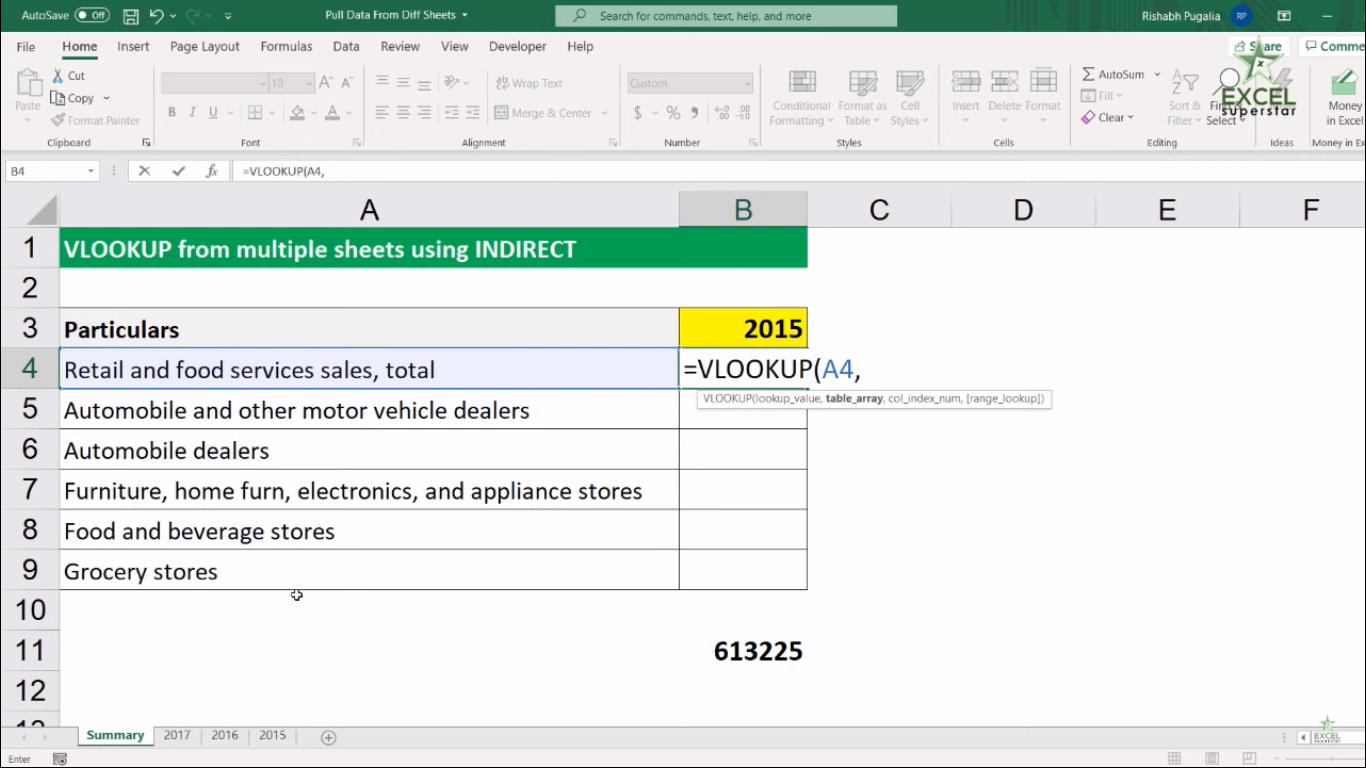The second parameter table_array means the table from which to retrieve a value.

3. Go to 2017 year and select the entire table =VLOOKUP(A4,’2017’!A:B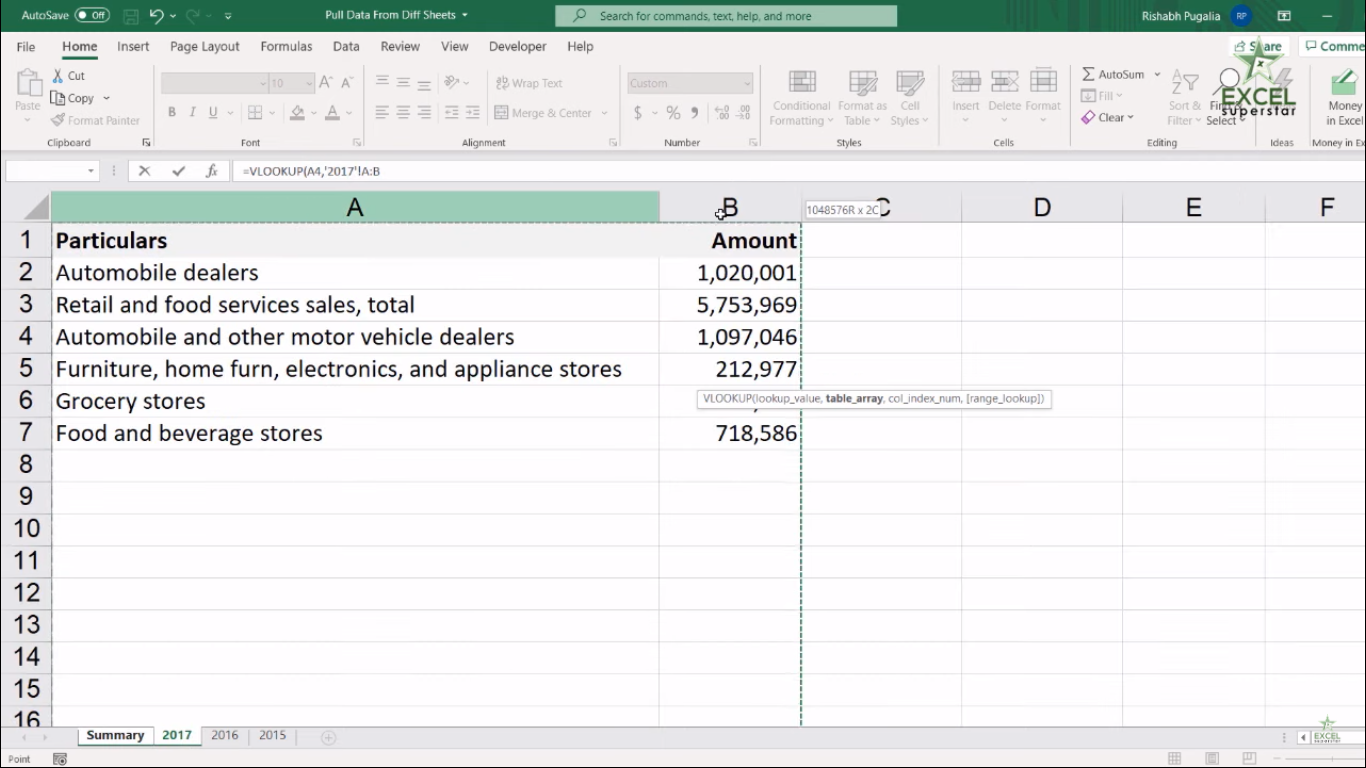The third parameter col_index_num means the column in the table from which to retrieve a value.

4. Write 2 =VLOOKUP(A4,’2017’!A:B,2,

The fourth parameter range_lookup means TRUE = approximate match and FALSE = exact match.

5. Write FALSE or 0 to get the answer as exact match =VLOOKUP(A4,’2017’!A:B,2,0

6. Close the bracket and press Enter =VLOOKUP(A4,’2017’!A:B,2,0)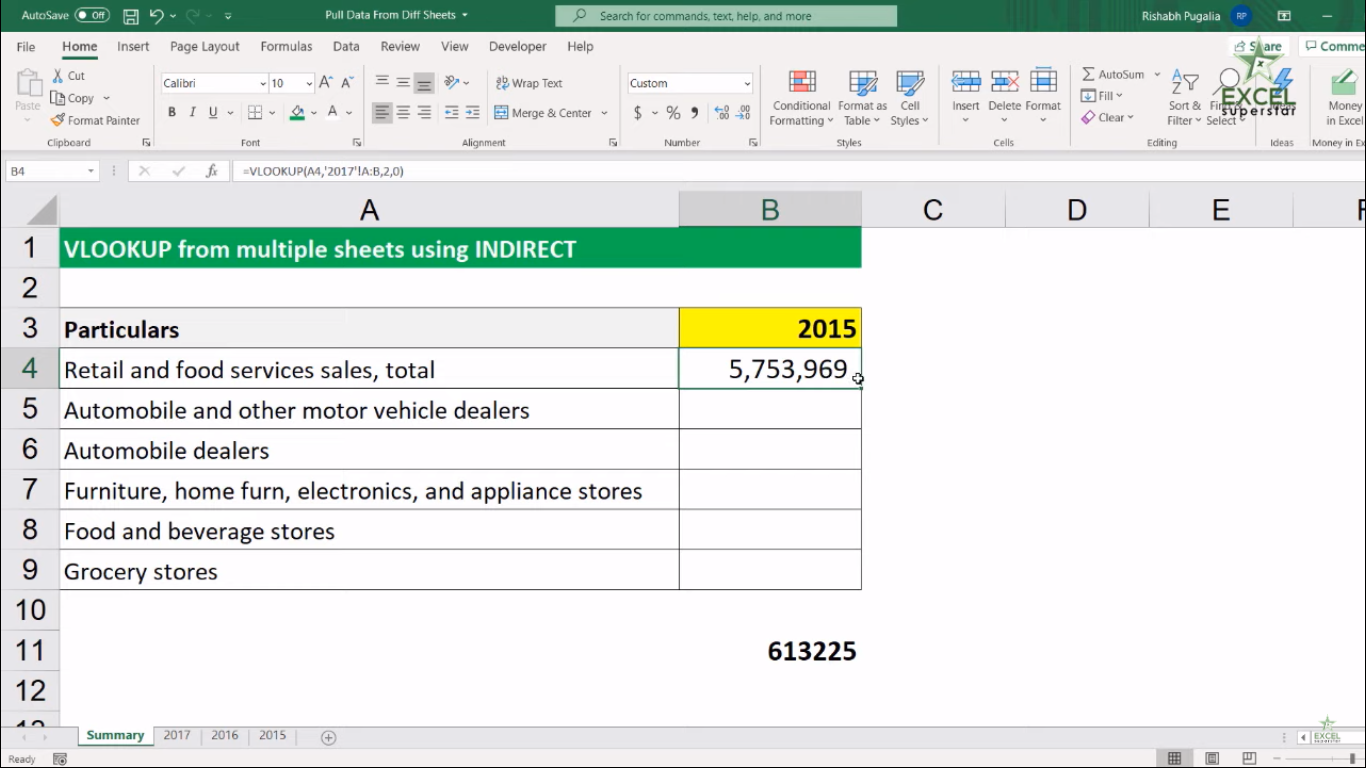7. You will get your answer for Retail and Food Services from the Sheet 2017

Now, if you change the year from 2017 to 2016 or 2015, then your answer will not change.

The reason behind not getting changed is that you are taking ‘’2017’!A: B sheet reference in the name of a table array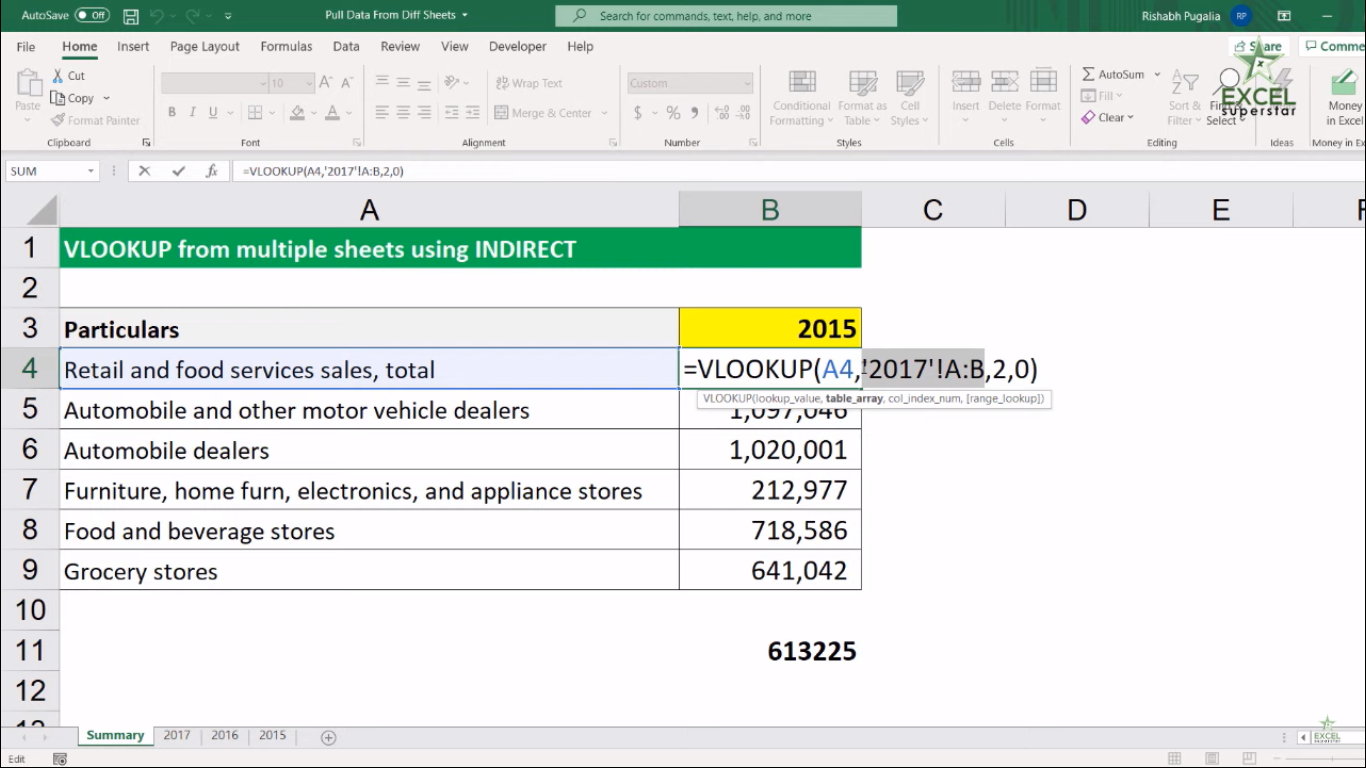So we want that in VLOOKUP Formula, when the table array comes, then it should change the reference on the basis of the yellow cell. In order to do this, follow the given steps below:

1. Copy the ‘’2017’! A: B sheet reference and paste it on another cell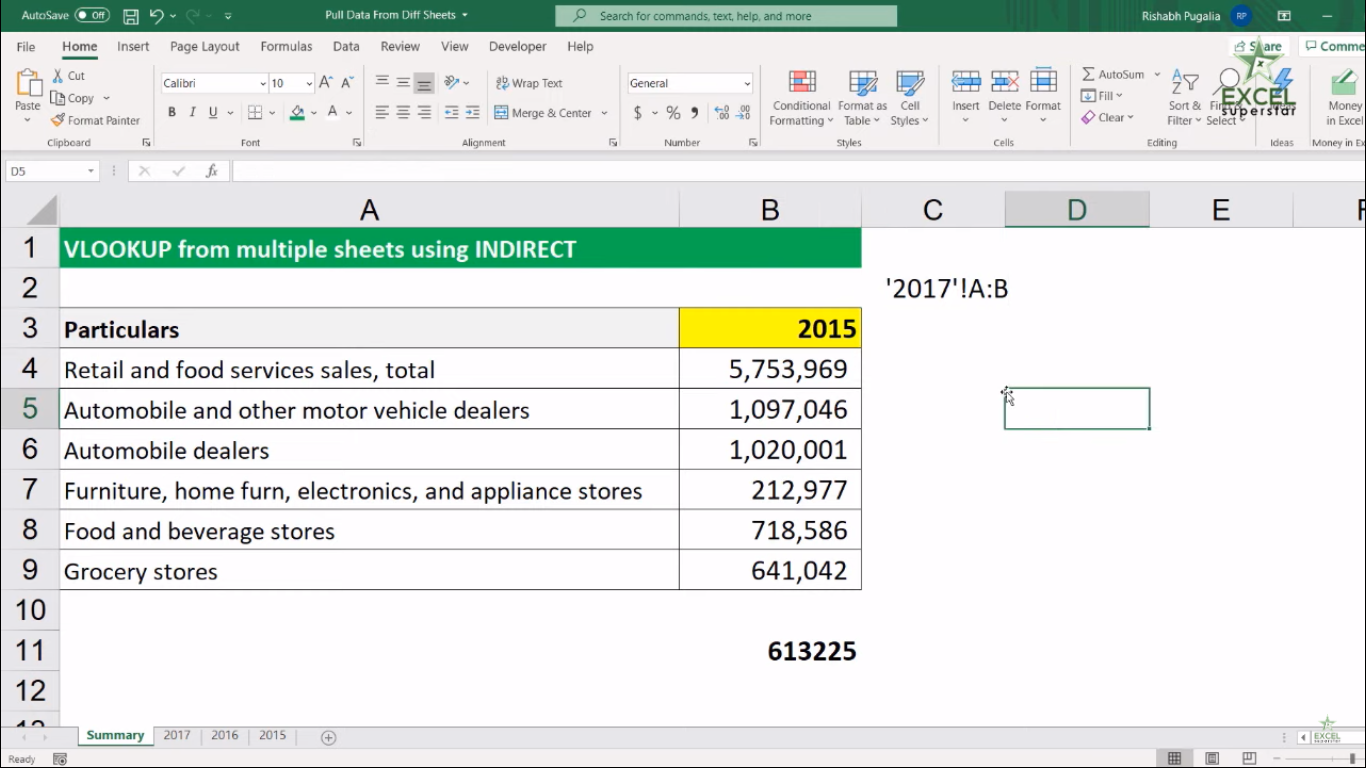Now instead of table_array we will be applying INDIRECT Formula

2. Write INDIRECT Formula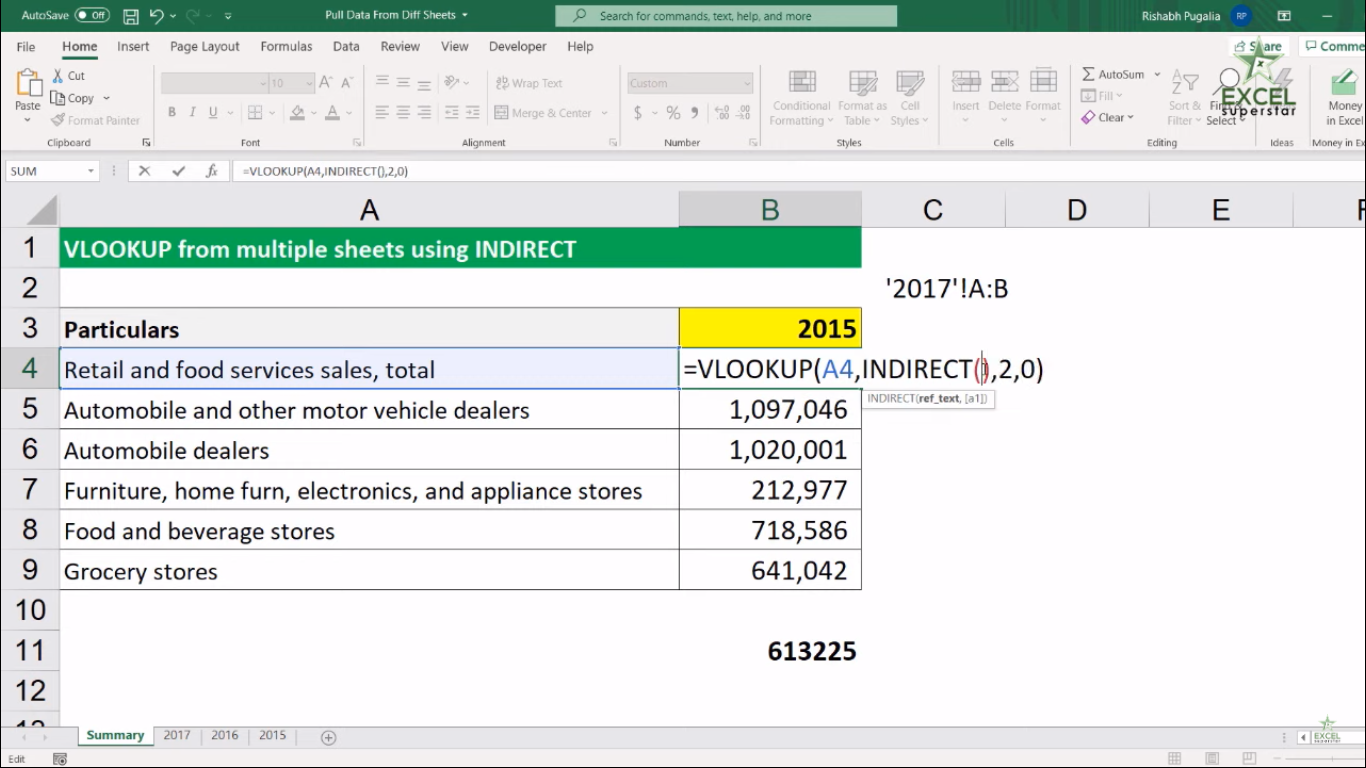3. Write Double Quotation (“) Single Quotation (’) and again Double Quotation (“) INDIRECT (”’”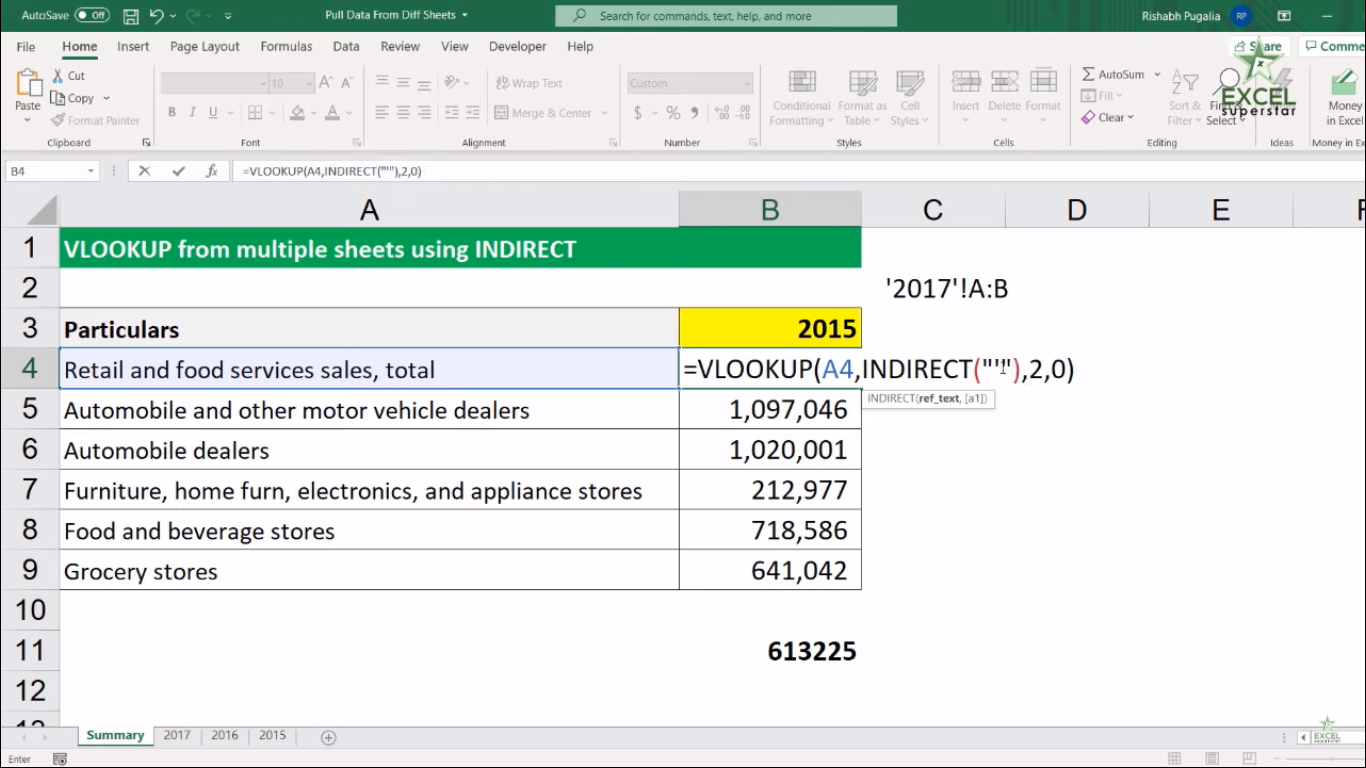4. Write Ampersand (&) INDIRECT (”’”&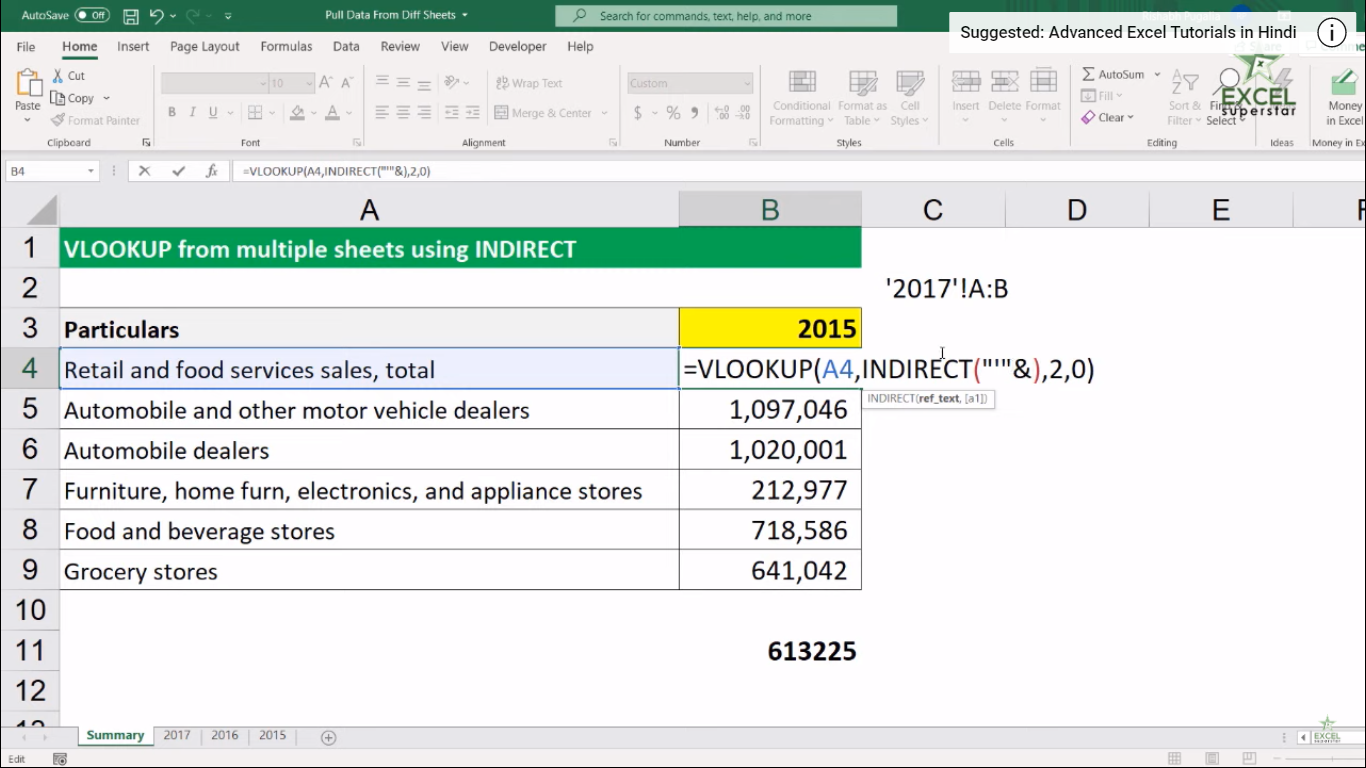5. Choose the yellow cell and press F4 INDIRECT (”’”&\$B\$3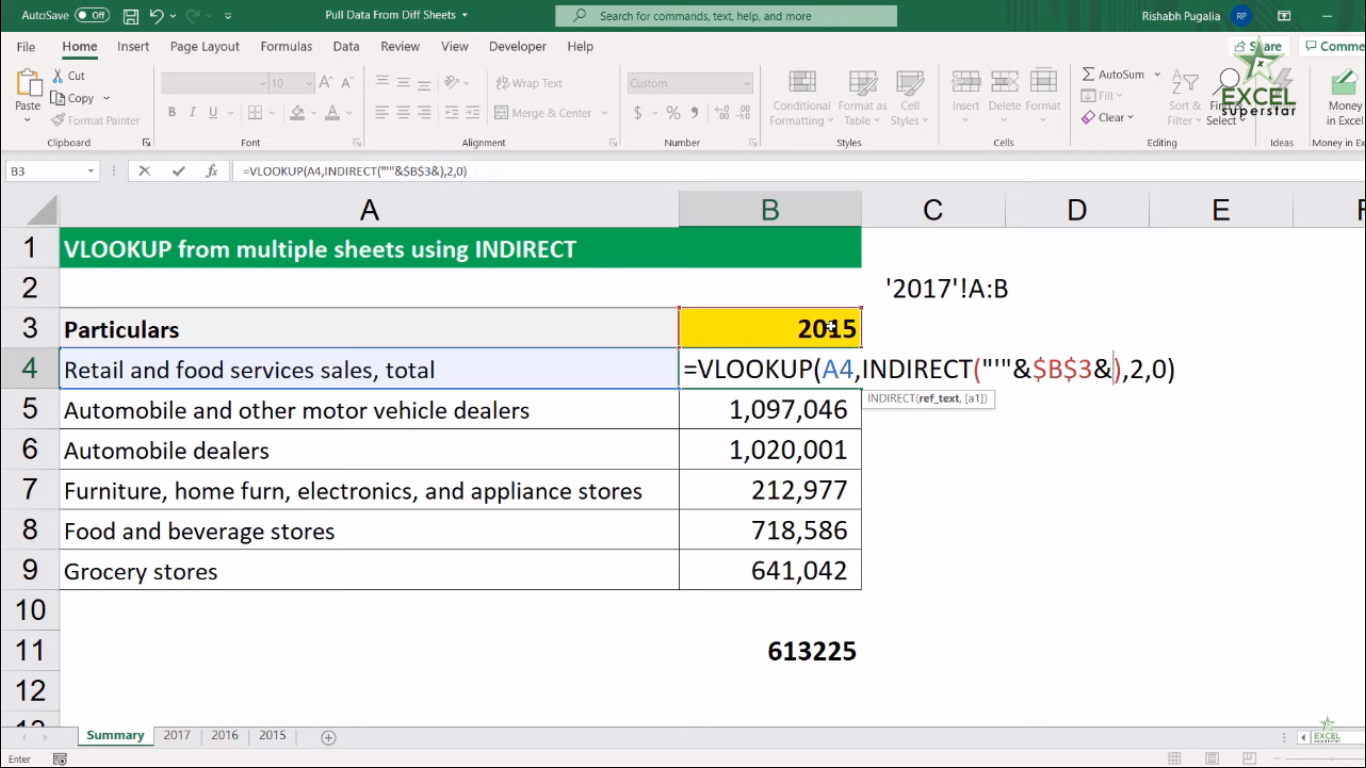6. Again write Ampersand (&) along with Single Quotation (’) and Double Quotation (“) INDIRECT (”’”&\$B\$3”’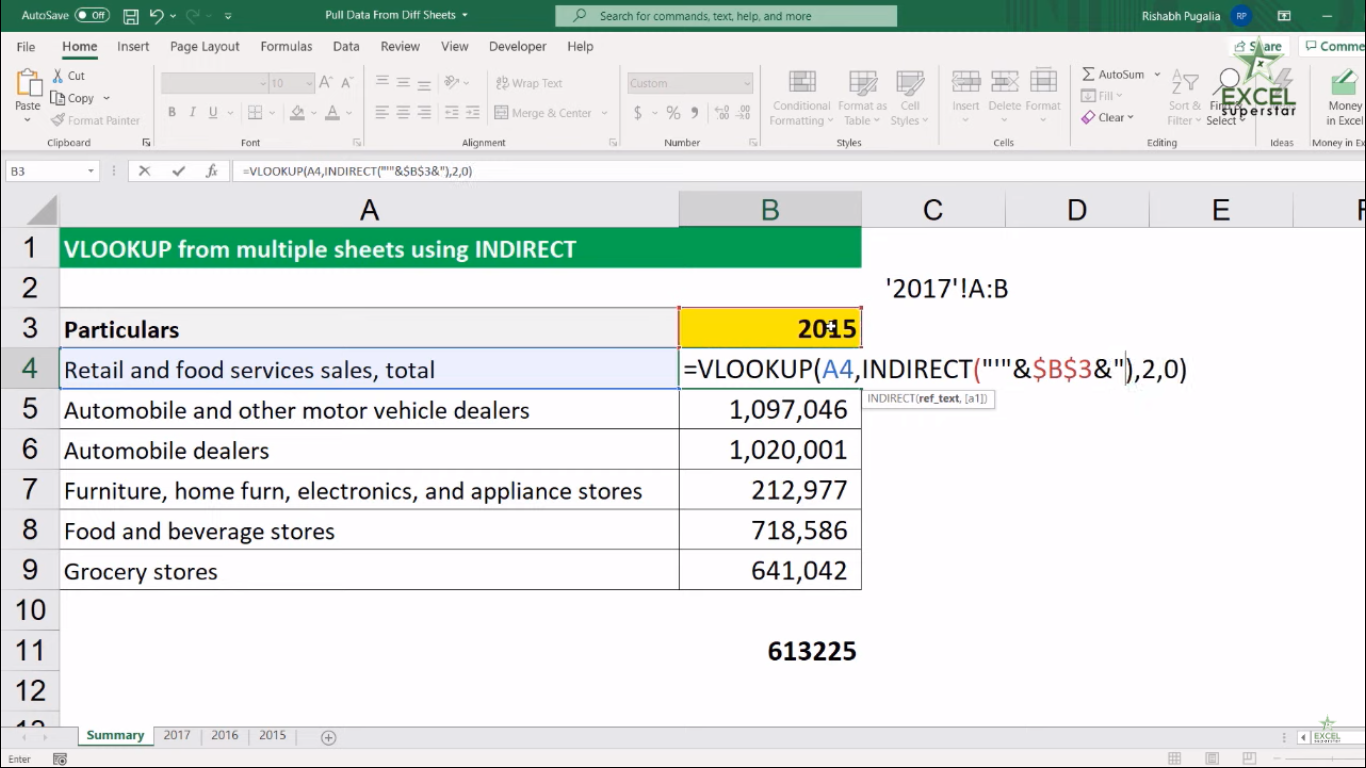7. Write A:B INDIRECT (”’”&\$B\$3”’A:B”